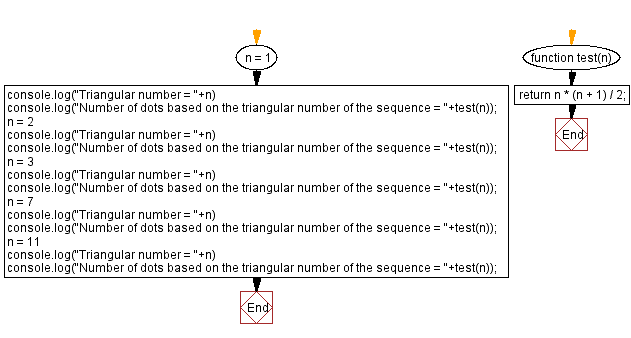# JavaScript: Sequence number from the given Triangular Number

## JavaScript Math: Exercise-79 with Solution

Write a JavaScript program to get the number of dots based on the triangular number of the sequence.

From Wikipedia -
A triangular number or triangle number counts objects arranged in an equilateral triangle. Triangular numbers are a type of figurate number, other examples being square numbers and cube numbers. The nth triangular number is the number of dots in the triangular arrangement with n dots on each side, and is equal to the sum of the n natural numbers from 1 to n. The sequence of triangular numbers, starting with the 0th triangular number, is
0, 1, 3, 6, 10, 15, 21, 28, 36, 45, 55, 66, 78, 91, 105, 120, 136, 153, 171, 190, 210, 231, 253, 276, 300, 325, 351, 378, 406, 435, 465, 496, 528, 561, 595, 630, 666...

Test Data:
(1) -> 1
(2) -> 3
(3) -> 6
(7) -> 28
(11) -> 66

Sample Solution:

HTML Code:

``````<!DOCTYPE html>
<html>
<meta charset="utf-8">
<title>JavaScript program to sequence number from the given Triangular Number</title>
<body>

</body>
</html>
```
```

JavaScript Code:

``````function test(n) {
return n * (n + 1) / 2;
}
n = 1
console.log("Triangular number = "+n)
console.log("Number of dots based on the triangular number of the sequence = "+test(n));
n = 2
console.log("Triangular number = "+n)
console.log("Number of dots based on the triangular number of the sequence = "+test(n));
n = 3
console.log("Triangular number = "+n)
console.log("Number of dots based on the triangular number of the sequence = "+test(n));
n = 7
console.log("Triangular number = "+n)
console.log("Number of dots based on the triangular number of the sequence = "+test(n));
n = 11
console.log("Triangular number = "+n)
console.log("Number of dots based on the triangular number of the sequence = "+test(n));
```
```

Sample Output:

```Triangular number = 1
Number of dots based on the triangular number of the sequence = 1
Triangular number = 2
Number of dots based on the triangular number of the sequence = 3
Triangular number = 3
Number of dots based on the triangular number of the sequence = 6
Triangular number = 7
Number of dots based on the triangular number of the sequence = 28
Triangular number = 11
Number of dots based on the triangular number of the sequence = 66
```

Flowchart:Live Demo:

See the Pen javascript-math-exercise-79 by w3resource (@w3resource) on CodePen.

Improve this sample solution and post your code through Disqus

What is the difficulty level of this exercise?

Test your Programming skills with w3resource's quiz.

﻿